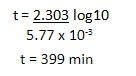Please check for Class 12 - Biology Important Questions

### List of Questions and Answers

1.The branch of chemistry, which deals with the study of reaction rates and their mechanisms, is called ______________.

Ans:Chemical Kinetics

2.What are the factors that affect the rate of reaction?

Ans: The factors affecting rate of reaction are as follows:

• Concentration
• Temperature
• Pressure
• Presence of Catalyst
• Surface Area

3.Define Rate of Reaction.

Ans: The rate of a reaction can be defined as the change in concentration of a reactant or product in unit time.

4.Define Rate Law.

Ans: The representation of rate of reaction in terms of concentration of the reactants is known as rate law. It is also called a rate equation or rate expression.

5.The rate constant for first order reaction is 60/s. How much time will it take to reduce the concentration of the reaction to 1/10 of its initial value.

Ans:
The first order reaction is :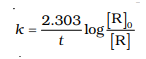We have rate constant k = 60/s, Ro = 1 and R = 1/10 and we have to find the time taken i.e t.
So the formula will be :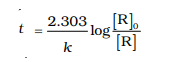Substituting the values we get :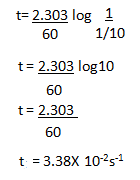6.The initial concentration of N2O5 in the following first order reaction N2O5(g) → 2NO2(g) + 1/2O2(g) was 1.24 × 10–2 mol L–1 at 318 K. The concentration of N2O5 after 60 minutes was 0.20 × 10–2 mol L–1. Calculate the rate constant of the reaction at 318 K.

Ans: The formula is :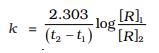We have t2 = 60 mins, t1 = 0 , R1 = 1.24 × 10–2 mol L–1 and R2 = 0.20 × 10–2 mol L–1.
After substituting the values we get :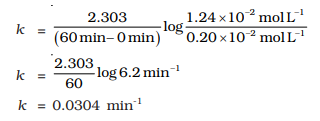7. (a) What is the order of reaction whose rate constant has the same unit as the rate of reaction?
(b) Thermal decomposition of a compound is of first order. 50% decomposes in 120 minutes. How long will it take for 90% to decompose?

Ans:
a) Zero order reaction.
b) The half life reaction to decompose takes 120mins
The formula is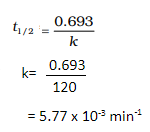Now applying first order reaction we have :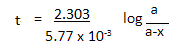We have a = 100 and x = 90 , so a-x = 10